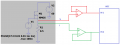# High voltage and current handling circuit

#### pinkyponky

Joined Nov 28, 2019
194
Hi,

I want to measure the voltage (upto 60V) and current (upto 30A) at the MOSFET. In such a way, the source terminal of the MOSFET are connecting to the input of the amplifier (to measure the parameters as voltage and current)-> output of amplifier is connecting to the ADC -> then connected to the MCU.

But, don't find the amplifiers and ADC's that are rated for above parameter range. So, please can you help me how to measure?. Do we need to design any circuits before the amplifier and ADC?.

#### BobTPH

Joined Jun 5, 2013
4,032
Show us the circuit and the places in it that you want to measure.

Bob

#### pinkyponky

Joined Nov 28, 2019
194
Show us the circuit and the places in it that you want to measure.

Bob
This is just starting stage of the circuit. I need to design from this stage only.

#### BobTPH

Joined Jun 5, 2013
4,032
Sorry, but my crystal ball is down right now.

How can we possibly answer a question about how to measure the voltage at the source of some MOSFET when we do not know how it is connected?

Okay, if you won't cooperate, I will make the most reasonable assumptiosn. In many (most?) circuits the source of an N-channel MOSFET is at ground. And N-channel ones are far more common the P-channel ones.

Therefore, my best guess is that the voltage at the source is 0.

Bob

#### pinkyponky

Joined Nov 28, 2019
194
Sorry, but my crystal ball is down right now.

How can we possibly answer a question about how to measure the voltage at the source of some MOSFET when we do not know how it is connected?

Okay, if you won't cooperate, I will make the most reasonable assumptiosn. In many (most?) circuits the source of an N-channel MOSFET is at ground. And N-channel ones are far more common the P-channel ones.

Therefore, my best guess is that the voltage at the source is 0.

Bob
Dear Bob,

Really, I don't have complete circuit to share with you. But, I have circuit which I have started just few hours ago. Yes, what you assume is right I have a n-channel MOSFET ( CSD19533KCS) that has been chosen. Can you help from here?.#### BobaMosfet

Joined Jul 1, 2009
1,897
Hi,

I want to measure the voltage (upto 60V) and current (upto 30A) at the MOSFET. In such a way, the source terminal of the MOSFET are connecting to the input of the amplifier (to measure the parameters as voltage and current)-> output of amplifier is connecting to the ADC -> then connected to the MCU.

But, don't find the amplifiers and ADC's that are rated for above parameter range. So, please can you help me how to measure?. Do we need to design any circuits before the amplifier and ADC?.
Generally speaking, scale the voltage down before measuring it. That's how you do that for any size voltage and current. Safety is paramount- and it reduces cost of components significantly while achieving same result. IMHO.

#### BobTPH

Joined Jun 5, 2013
4,032
The two measurements you show are both measuring the voltage across the the resistor, which is proportional to the current.

To scale that value, you simply choose the value if the resistor to put out the desired voltage at the max current.

No idea what voltage you are trying to measure.

Bob

#### dcbingaman

Joined Jun 30, 2021
495
Are you trying to measure the drain side voltage of M1? Think about the fact that M1 is driven on by a Gate to Source voltage. In this case you have negative feedback that will limit the current. How much depends entirely on the part number of the MOSFET. If you are trying to measure the voltage at the source, then the current and the voltage can be checked with one channel. Can you be more exact about the requirements?

#### dcbingaman

Joined Jun 30, 2021
495
What is the voltage range of the ADC? This plays a critical role in scaling things properly so we are within the range of the ADC. Without knowing that it is difficult to provide an answer. Also your circuit cannot have more than the gate voltage on the source. In fact it will always be less by whatever is required to bring the MOSFET into conduction. Assume the MOSFET needs 2V minimum between the gate and the source to turn 'on'. You will see 3V in that case on the source terminal and the max current of that circuit is just that voltage divided by R1.